# Quick Overview of Convolutional Neural Networks across 4 Frameworks — TensorFlow, Keras, Chainer…

Source: Deep Learning on Medium

Deep Learning Framework:

we will go through the brief description of 4 Deep Learning Frameworks and the frameworks under discussion here are: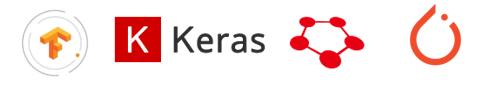Frameworks

TensorFlow:

TensorFlow™ is an open source software library for high performance numerical computation . Its flexible architecture allows easy deployment of computation across a variety of platforms (CPUs, GPUs, TPUs) .

Keras:

Keras is a high-level neural networks API, written in Python and capable of running on top of TensorFlow, CNTK, or Theano. It was developed with a focus on enabling fast experimentation .

Chainer:

A Python framework to quickly implement, train and evaluate deep learning models. It follows Define-by-Run approach .

PyTorch:

It’s a Python-based scientific computing package targeted at two sets of audiences: 1) A replacement for NumPy to use the power of GPUs 2) A deep learning research platform that provides maximum flexibility and speed .

Convolutional Neural Networks:

What is Convolution?

Convolution Operation is a fundamental building block of CNN. It is a mathematical operation on two different matrices to output a new matrix based on above 2 matrices. It basically identifies the new shape based on the operation performed. This new shape identified might find vertical edges or horizontal edges of the image depending on what kind of filter is selected. Below Figures briefly explains how convolution operation works: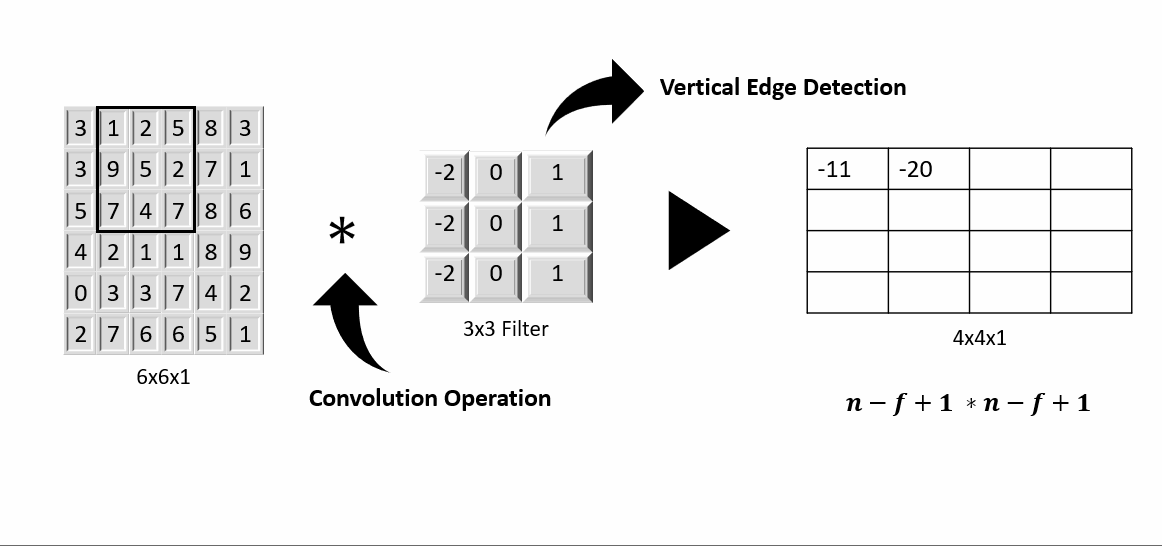Figure: Convolution Operation for Vertical Edge Detection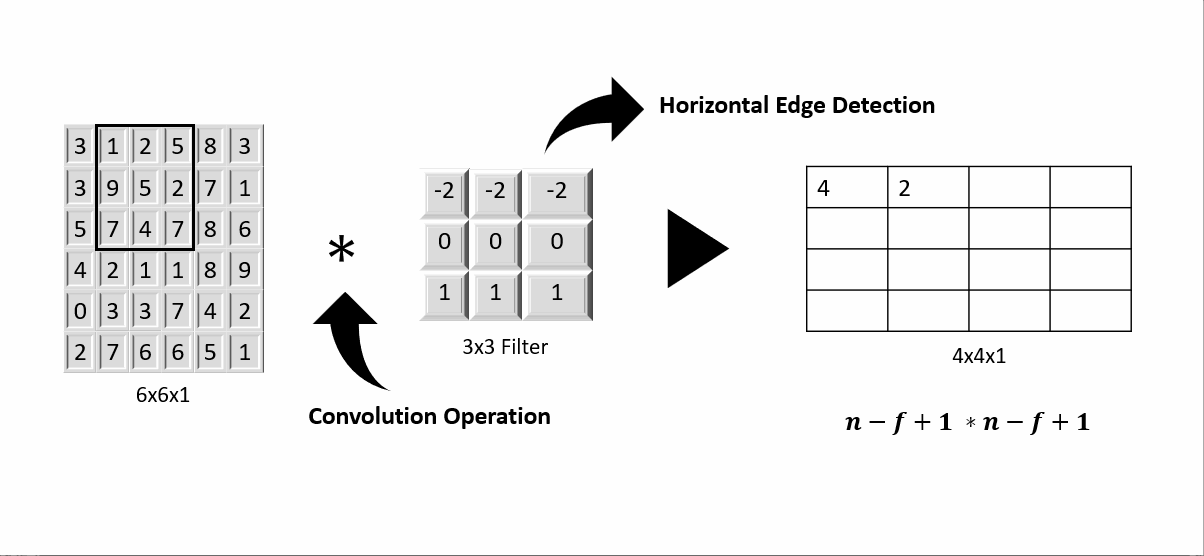Figure: Convolution Operation for Horizontal Edge Detection

However, during the process of Convolution Operation either for Vertical Edge detection or Horizontal Edge detection leads to shrinking in output size. In order to avoid this shrinking of output another hyperparameter is defined which is termed as Padding. Based on the number defined for Padding, It adds that many number of borders to the input which is basically an image. Thus, when convolution operation is performed to such input with the filter of choice then the shape of the output resulted will be equal to the original size of the input without the borders added by padding. Below Figure briefly explains the importance of padding.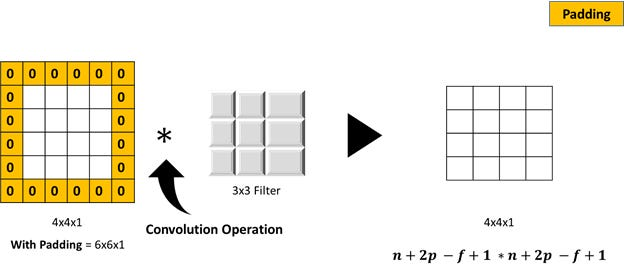Figure: Convolution Operation with Padding implemented

There are two types of Padding Operations: 1) Valid Convolution and 2) Same Convolution. “Valid” Convolution means no padding and the resulted output size will not match with the input size and “Same” Convolution means there is padding greater than 0 and in this case the size of the output is same as the input size.

Another hyperparameter which plays prominent role during Convolution operation is “Strides”. Strides is nothing, but the no of steps convolution operation will consider either horizontally or vertically thus further increasing the pixels between samples. Below Figure briefly explains the striding process.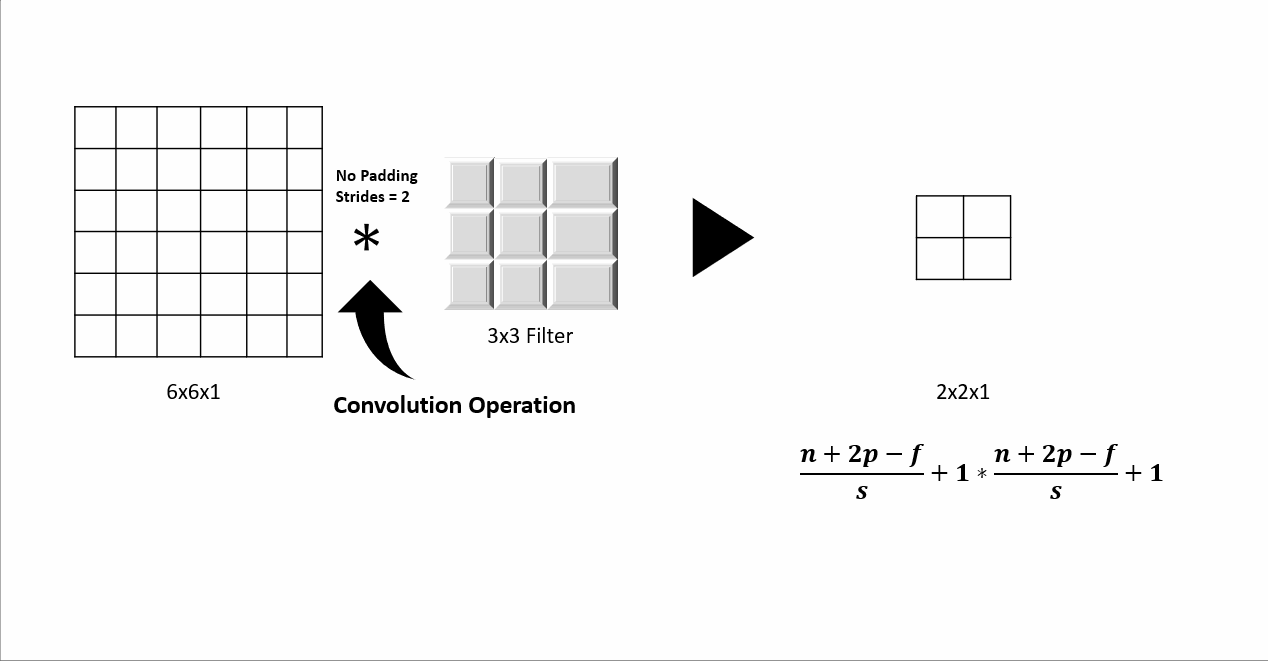Figure: Convolution Operation with Strides

We have designed our Convolutional Neural Network based on one of the most common classic networks “ResNets” i.e. CONVOLUTIONAL 2D LAYER >> MAX-POOLING LAYER >> CONVOLUTIONAL 2D LAYER >> MAX-POOLING LAYER >> FLATTEN LAYER >> FULLY CONNECTED LAYER >> FULLY CONNECTED LAYER >> SOFTMAX. Below is the diagrammatic representation of the neural network under discussion.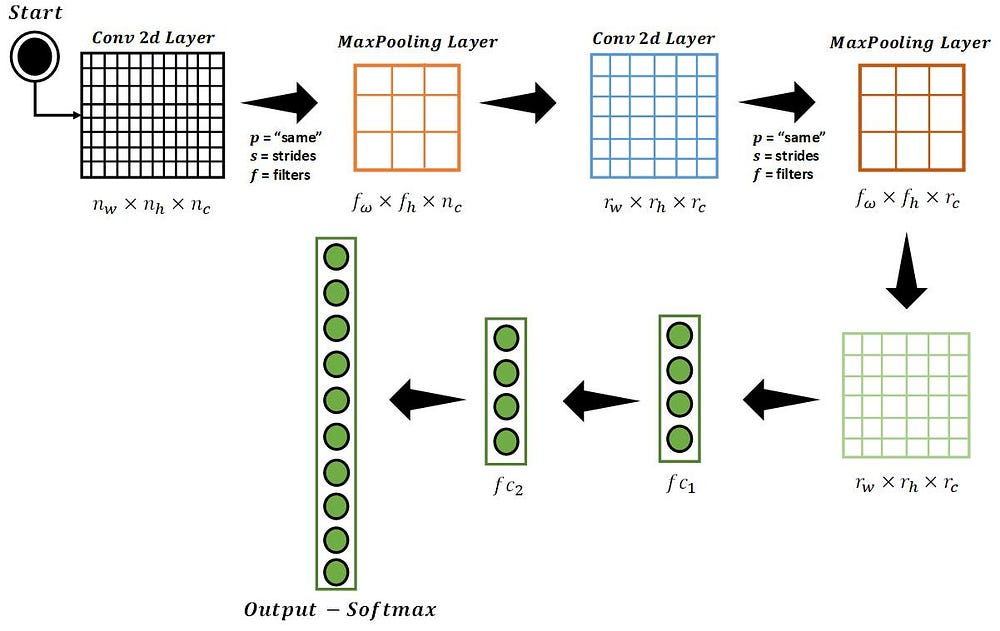Figure 1: A quick overview Convolutional Network being used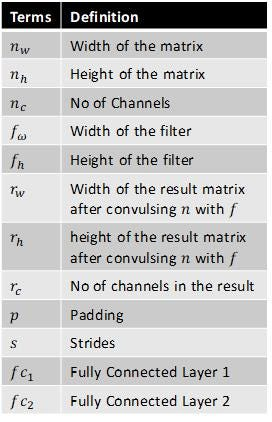Definition of Terms in Figure 1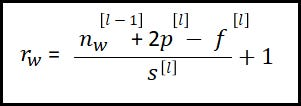Formula for width of the result matrix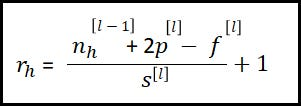Formula for height of the result matrix

As we see in Figure 1, there are two layers which are called as MaxPooling layer but what are these MaxPooling layers? Before understanding about this layer lets first understand why we need Pooling layer. Pooling layer is needed to reduce the size of the output thus reducing the number of parameters which in turn reduces the computation and speed up the learning of the network. There are two basic types of Pooling Layers: 1) Max Pooling and 2) Average Pooling. Max Pooling layer based on its filter size during the convolution operation picks the max number of all the cells in scope and insert into the desired cell of the output and so on whereas Average Pooling takes the average of all the cells involved and insert that new value into the desired cell of the output. Since we are following “ResNets” framework design we have considered MaxPooling layer. Below Figures briefly explains the process of MaxPooling and AveragePooling.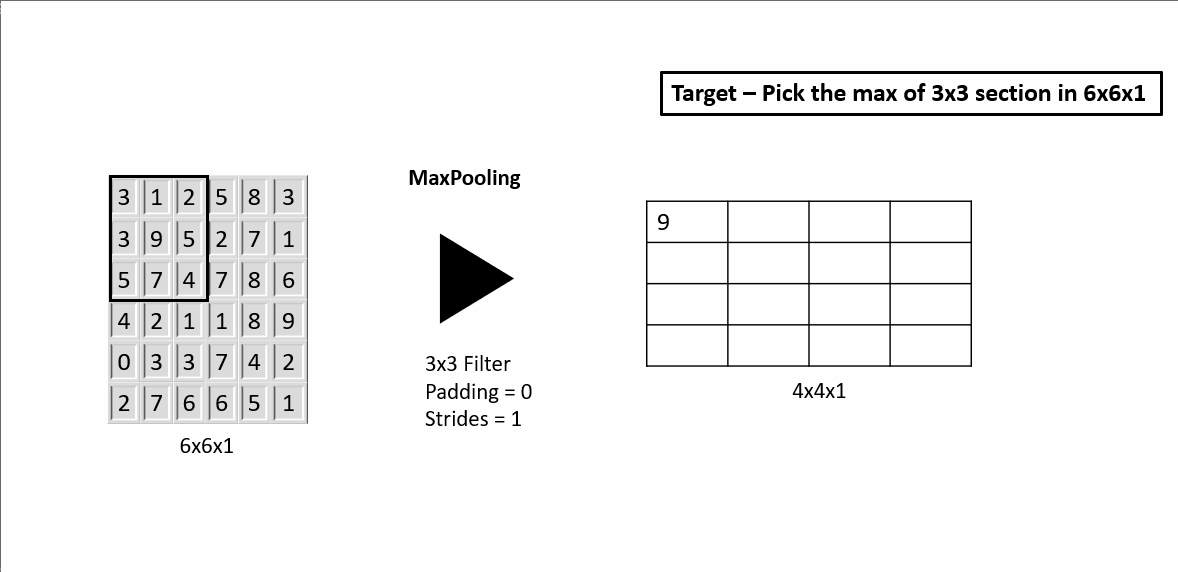Figure: How MaxPooling Works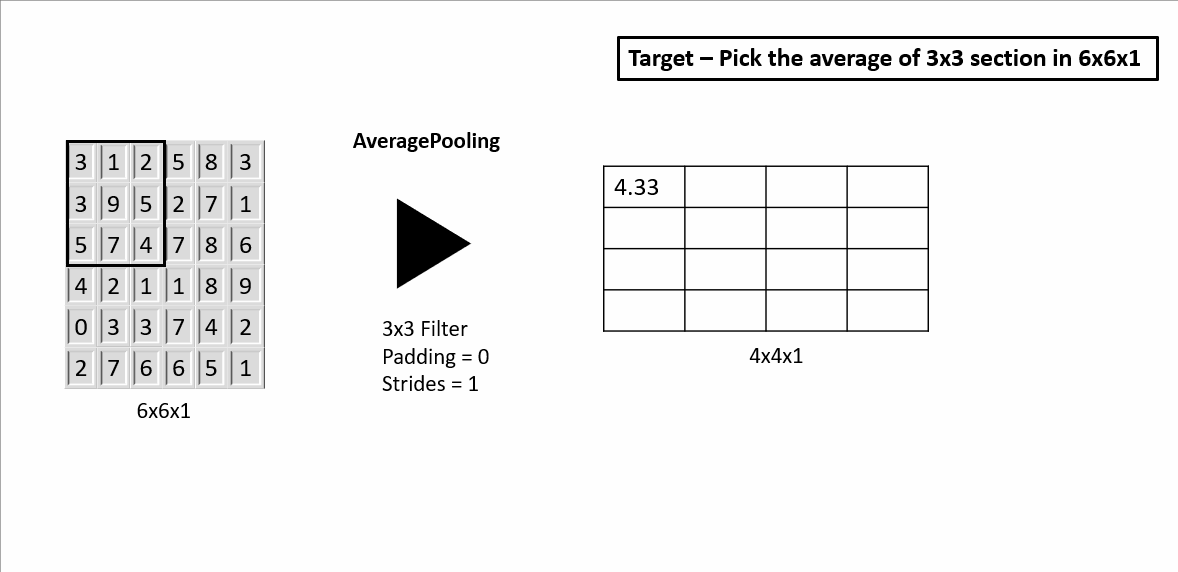Figure: How AveragePooling Works

“Same” Convolution operation is performed on the input with the filter of appropriate size and padding. Once the new output and activation function such as “ReLU” is applied to that output before transferring that as an input to the Max Pooling layer. Based on the filters and strides defined, MaxPooling is applied to the input. Once completed this output serves as an input for the convolutional 2D layer which in turn repeats the process until the layer where the output is flattened into one-hot vector which is basically multiplying height * width * channels and showing that many numbers of rows. After flattening the output, that new structure serves as an input to the fully connected layer, the layer where each node is connected to every row of the input. The result of this layer serves as an input to another fully connected layer till the final layer is reached where “SOFTMAX” activation function is applied to generate 1 of 10 probable’s which in this case are the numbers from 0–9.

Implementation of Convolutional Neural Network

TensorFlow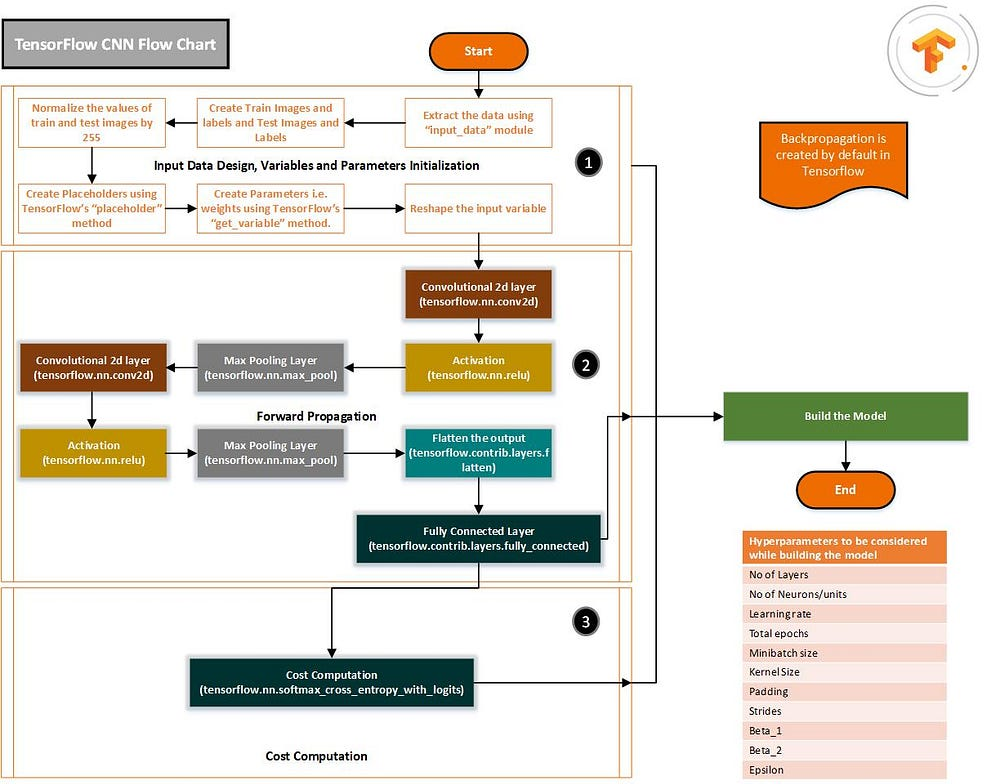1.1 Convolutional Neural network in TensorFlow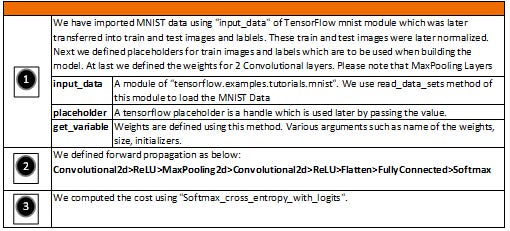Glossary for Figure 1.1

Keras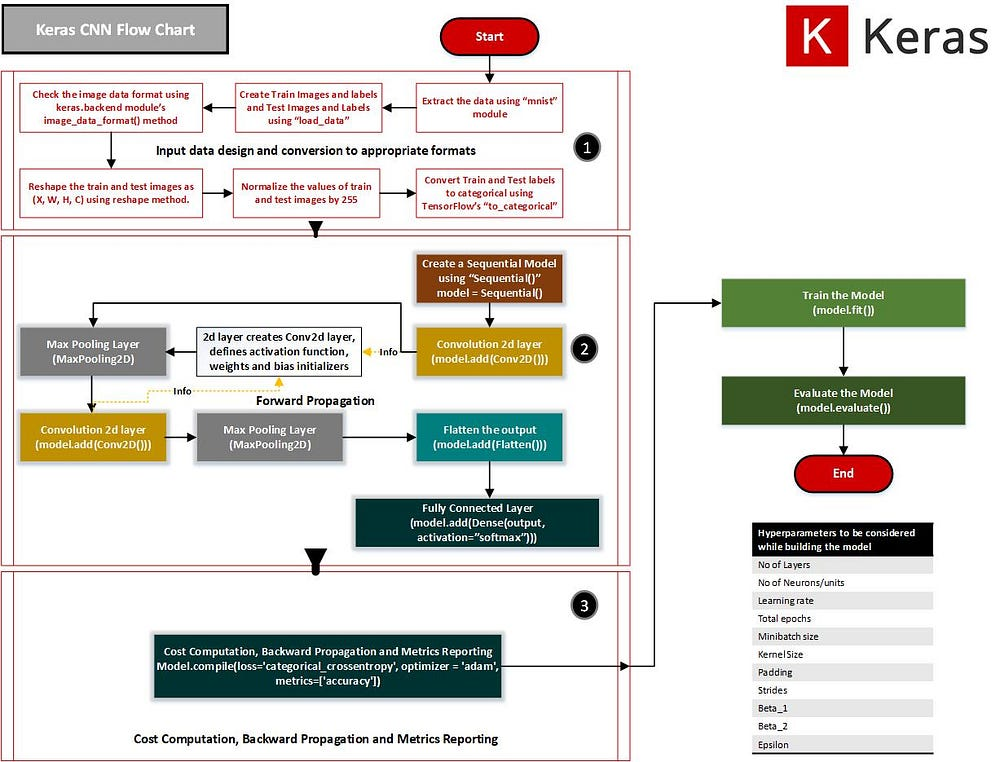1.2 Convolutional Neural Network in Keras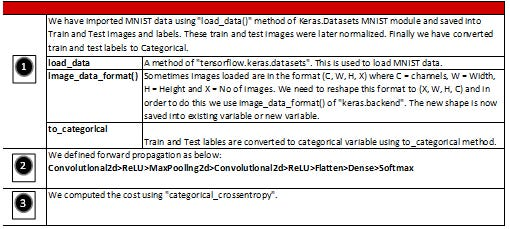Glossary for Figure 1.2

Chainer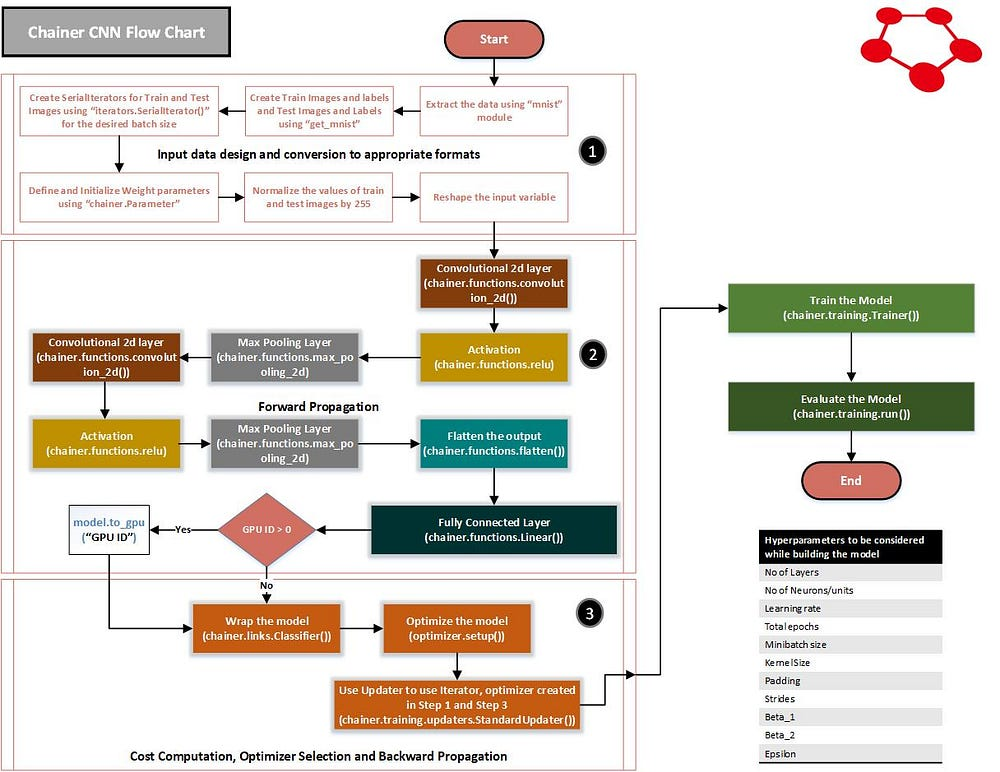1.3 Convolutional Neural Network in Chainer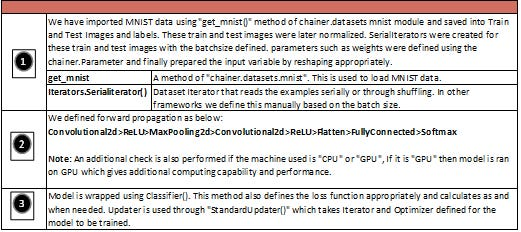Glossary for Figure 1.3

PyTorch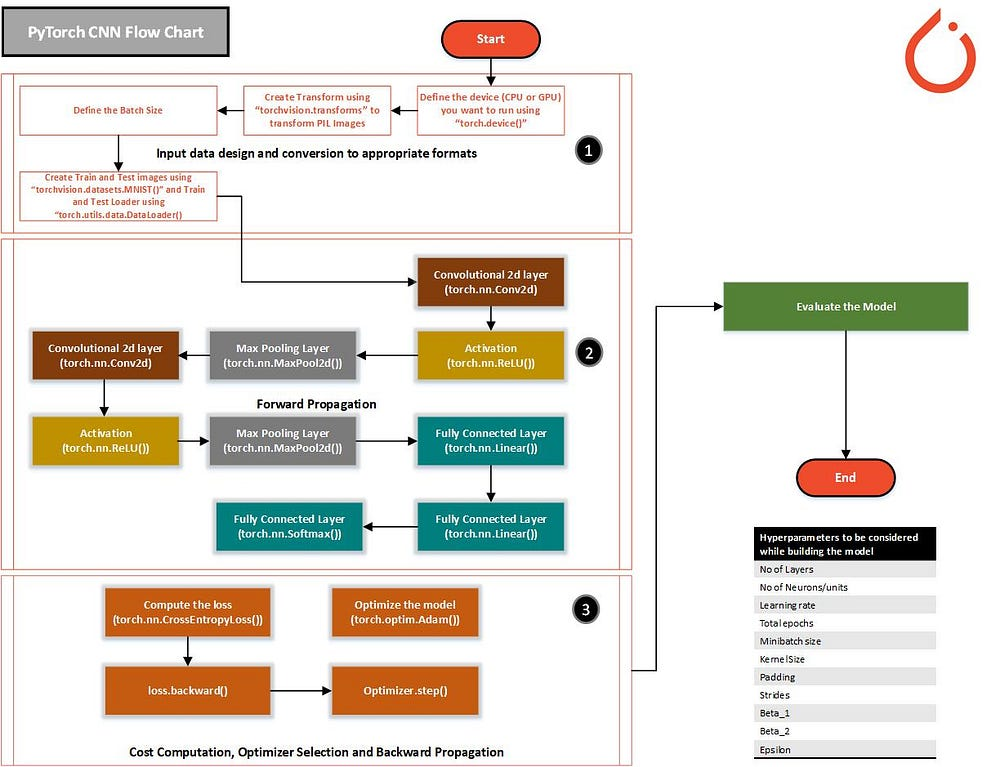Convolutional Neural Network in PyTorch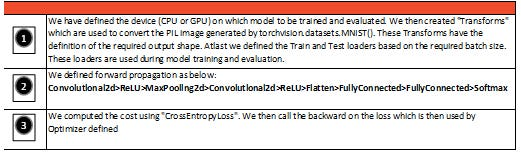Glossary for Figure 1.4